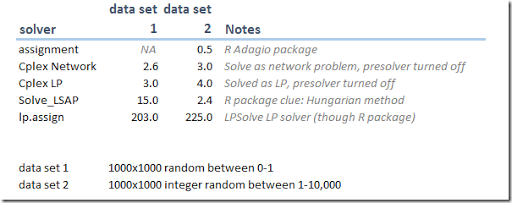## Wednesday, March 16, 2016

### Large assignment problem

I usually solve assignment problems:

 \begin{align} \min\>& \sum_{i,j} c_{i,j} x_{i,j} & \\ & \sum_j x_{i,j} = 1 & \forall i \\ & \sum_i x_{i,j} = 1 & \forall j \\ & x \in \{0,1\} \end{align}

as an LP or MIP. It is often argued that specialized solvers are much faster on this. Here some results with a $$1000\times 1000$$ problem, artificially created:See also here. One of the advantages of using an LP/MIP is that I can react more quickly on changes in the problem. Often what starts out as a straight assignment problem becomes in the end something rather different. See here for an example of that.

Notes:

• The assignment solver in the R Adagio package deals with integer cost coefficients only.
• Current state-of-the art LP solvers are very, very fast. The need for specialized network solvers in these packages becomes somewhat less apparent. Indeed Gurobi does not offer one.
• lp.assign is just calling the LPSolve LP/MIP solver.